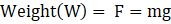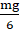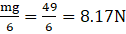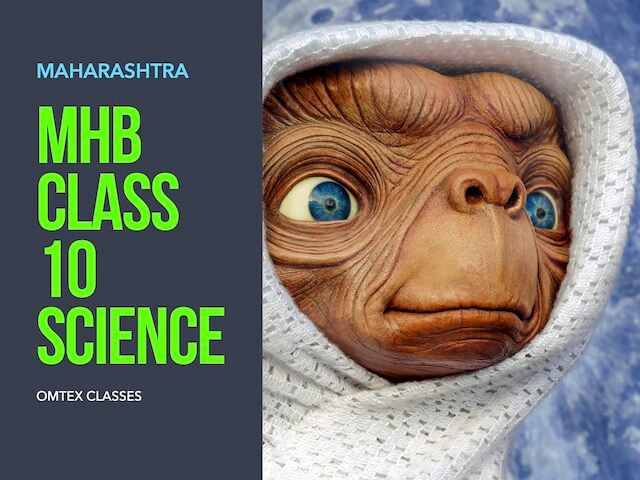### Question 11. Solve the following example. The mass and weight of an object on earth are 5 kg and 49 N respectively. What will be their values on the moon? Assume that the acceleration due to gravity on the moon is 1/6th of that on the earth.

Question 11.

Solve the following example.

The mass and weight of an object on earth are 5 kg and 49 N respectively. What will be their values on the moon? Assume that the acceleration due to gravity on the moon is 1/6th of that on the earth.

Mass value: Since mass is the amount of matter present in our body and it remains same irrespective of the change in position. Therefore the value of mass will be 5 Kg on moon as well.

Weight value: Weight is the force with which an object is attracted by a planet and it is equal to;Where m = mass of the object;

g = acceleration due to gravity;

On earth weight(W1) = mg = 49N;

On moon weight(W2) =(acceleration due to gravity is one-sixth of earth).

Hence weight on moon =Therefore Mass on moon is 5kg and weight on moon is 8.17N.